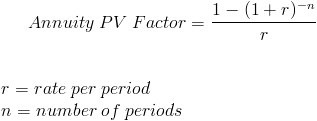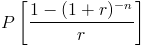# Present Value Annuity FactorThe present value annuity factor is used to calculate the present value of future one dollar cash flows.

This formula relies on the concept of time value of money. Time value of money is the concept that a dollar received at a future date is worth less than if the same amount is received today. An amount received today can be invested towards future earnings or receive sooner utility. For this particular formula, the present value of one dollar periodic cash flows is to be used for simplifying the calculation of payments larger than one dollar. An example of this equation in practice is determining the original amount of a loan.

## Use of Present Value Annuity Factor Formula

The present value annuity factor is used for simplifying the process of calculating the present value of an annuity. A table is used to find the present value per dollar of cash flows based on the number of periods and rate per period. Once the value per dollar of cash flows is found, the actual periodic cash flows can be multiplied by the per dollar amount to find the present value of the annuity.

For example, an individual is wanting to calculate the present value of a series of \$500 annual payments for 5 years based on a 5% rate. By looking at a present value annuity factor table, the annuity factor for 5 years and 5% rate is 4.3295. This is the present value per dollar received per year for 5 years at 5%. Therefore, \$500 can then be multiplied by 4.3295 to get a present value of \$2164.75.

## How is the Present Value Annuity Factor Formula Derived?

The present value annuity factor can be found by looking at the complete formula for the present value of an annuity:The payment variable can be taken out of the formula to determine the factor. By having a table that consists of the various factors associated with given rates and periods, calculating the present value of annuity can be simplified.

New to Finance?